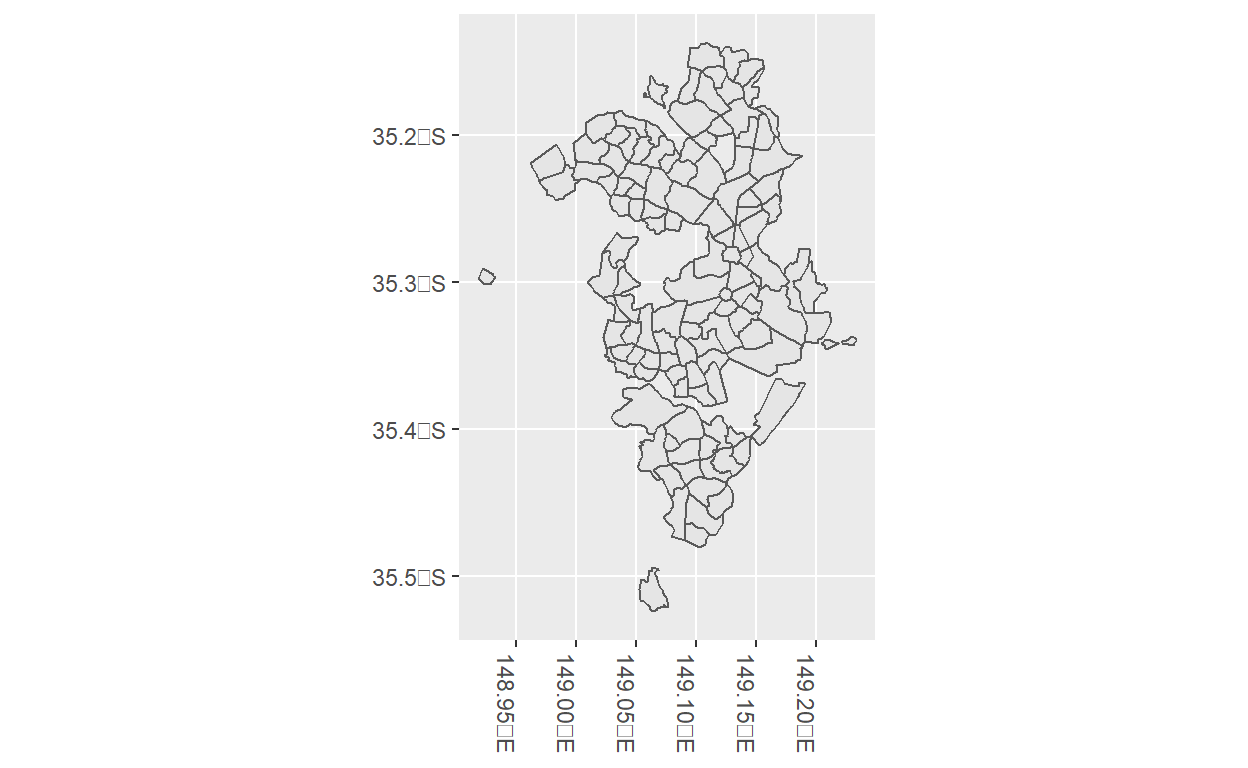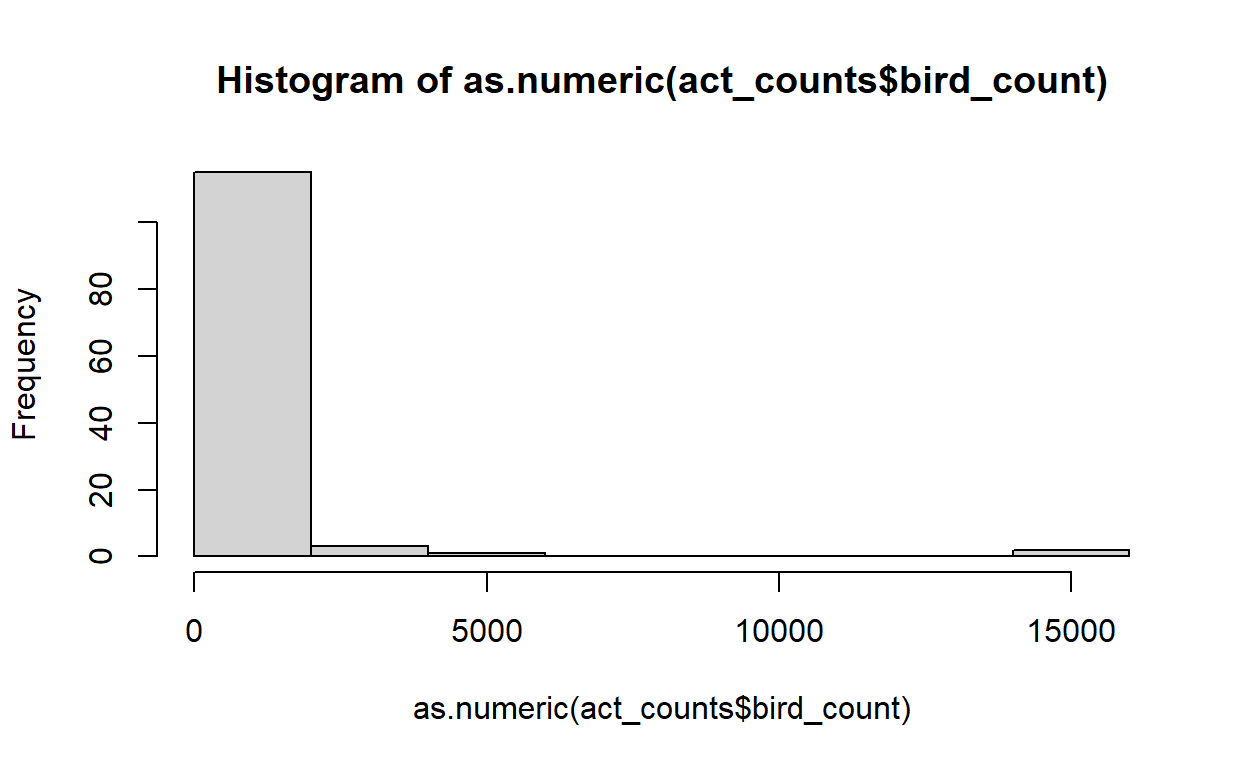# Counting points in multipolygon shapefiles for choropleth mapping

Choropleth maps are an excellent way to visualise numbers of observations, but when using point data, calculating the number of points in each polygon can be difficult when using shapefiles. Here we demonstrate how to extract and summarise the number of points in each polygon to create a choropleth map.

Olivia Torresan , Dax Kellie
2023-01-12#### Date:

12 January, 2023

Choropleth maps are an excellent way to visualise differences in variables (eg. number of species observed) across several geographic regions (eg. countries, states, study areas). Often, creating a choropleth map from species observations requires two things:

1. A shapefile - a file with vector data of a specific geographic location, with detailed info of its geographic features
2. Species observations recorded as point data - the specific longitude and latitude coordinates of where an individual was observed.

However, to create a choropleth map of species observations requires us to summarise our points to a single statistic for each polygon of our shapefile. This conversion from points to polygons can sometimes be tricky!

Here, we show you how to extract and count the number of points inside each polygon of a shapefile to create a choropleth map of the total number of species observations in each suburb of the Australian Capital Territory (ACT).

First we will load the R packages that we need:

library(feathers)
library(galah)
library(here)
library(rmapshaper)
library(tidyverse)
library(sf)

Next we will need a shapefile. You can find many shapefiles online from reputable sources. For this example, I’ve downloaded a shapefile of suburb boundaries in the city of Canberra, ACT from the ACT’s open-access map database.

Usually when you download a shapefile, it is compressed within a zip folder. Save this downloaded zip folder in a local folder inside your current R project. If you need to unzip your folder, you can do so with the following code:

zip_folder <- here("folder-name", "shapefile-folder-name.zip")
output_dir <- "folder-name-to-save-unzipped-files"
unzip(zip_folder, exdir = output_dir) 

Now we load this unzipped shapefile into R. To save space, we’ll remove some complexity from our shapefile polygons with ms_simplify() from the {rmapshaper} package.

The actsuburbs shapefile contains both suburb boundaries and “district” boundaries, which can encompass several suburbs. To avoid confusion, we will remove districts using filter(LOC_CLASS != "District"). We’ll also use st_make_valid() to make sure any weird invalid geometries in our shapefile are made valid, and therefore plot correctly.

actsuburbs <- st_read(here("folder-name",
"folder-name-2",
"shapefilename.shp") |>
ms_simplify(keep = 0.1) |>
st_transform(crs = st_crs("WGS84")) |>
st_make_valid() |>
filter(LOC_CLASS != "District")

Now to see if our shapefile plots correctly, we can use geom_sf() (and it looks like it does!)

ggplot() +
geom_sf(data = actsuburbs) +
theme(axis.text.x = element_text(angle = -90, hjust = 0))Next let’s use the {galah} package to download bird occurrence records from the Atlas of Living Australia (ALA).

We can download all Aves (bird) data provided by BirdLife Australia within the ACT by using galah_filter() to narrow our download. We’ll also add ALA’s data profile, or what the ALA calls a set of data quality filters to remove suspicious records, using galah_apply_profile(ALA).

You will need to provide a registered email with the ALA using galah_config() before retrieving records.

galah_config(email = "your-email@email.com", verbose = FALSE) 
birdocc <- galah_call() |>
galah_identify("Aves") |>
galah_apply_profile(ALA) |>
galah_filter(stateProvince == "Australian Capital Territory",
dataProviderName == "BirdLife Australia") |>
atlas_occurrences() 

## Count points in each polygon

To prepare our data, we’ll convert each observation into a format suitable for spatial mapping. st_as_sf() transforms each point into an sf spatial object (which plots nicely with {ggplot2}). We’ll also make sure the points are projected to crs = set_crs("WGS84"), the same as our shapefile, so that the points line up correctly.

bird_points_sf <- birdocc |>
st_as_sf(coords = c("decimalLongitude", "decimalLatitude"),
crs = st_crs("WGS84"))

Now we’ll find and count how many points are in each of our suburbs.

The st_intersects() function checks whether each point is within, or “intersects”, a specified POLYGON and then marks it as TRUE or FALSE in a matrix. Using st_intersects() in a loop with pmap() allows us to run st_intersects() on each row of a supplied list.

In our case, because each row of actsuburbs$geometry corresponds to each suburb, pmap() recursively checks which points are within each of our ACT suburbs! Adding lengths() around st_intersects() will count the number of rows returned for each suburb list, returning the total number of points that intersect each suburb. 1. We’ve saved this count in a new column bird_count. Note: This function takes ~3.5 minutes to run act_counts <- actsuburbs |> mutate(bird_count = pmap(.l = list(x = actsuburbs$geometry),
.f = function(x) {
lengths(st_intersects(x, bird_points_sf))
}))

act_counts |> select(LOC_NAME, bird_count) |> head(8L) # see sample of counts
Simple feature collection with 8 features and 2 fields
Geometry type: POLYGON
Dimension:     XY
Bounding box:  xmin: 149.0563 ymin: -35.48032 xmax: 149.2188 ymax: -35.15856
Geodetic CRS:  WGS 84
LOC_NAME bird_count                       geometry
1     Acton        670 POLYGON ((149.128 -35.28592...
2   Ainslie        120 POLYGON ((149.1374 -35.2583...
3    Amaroo         50 POLYGON ((149.1157 -35.1682...
4    Aranda         38 POLYGON ((149.0876 -35.2551...
5     Banks          0 POLYGON ((149.1019 -35.4803...
6    Barton        755 POLYGON ((149.1295 -35.3070...
7     Beard          0 POLYGON ((149.2156 -35.3407...
8 Belconnen       1521 POLYGON ((149.0789 -35.2304...

If we check our bird counts, we can notice that our count data is skewed because many regions have lower numbers of observations and only a few regions have very high numbers of observations.

hist(as.numeric(act_counts\$bird_count))Log transformation will reduce the skew in our data, ultimately making our final choropleth map easier to interpret.

Let’s add log-transformed counts to a new column log_counts. Next we’ll add a column called counts_discrete and use cut() to assign a number from 0 to 5 depending on what range each log_counts number falls within (0-1, 1-2, 2-3, 3-4 or 4-5). To make this easier to interpret, we’ve added labels that correspond to the un-transformed values (0, 10, 100, 1000, 10000). Finally, we replace any pesky NA values with 0.

act_counts <- act_counts |>
rowwise() |>
mutate(log_counts = log10(bird_count)) |>
mutate(counts_discrete = cut(log_counts,
breaks = c(0, 1, 2, 3, 4, 5),
labels = c(0, 10, 100, 1000, 10000),
include.lowest = TRUE)) |>
replace_na(list(counts_discrete = "0"))

act_counts |> rmarkdown::paged_table() # final data frame